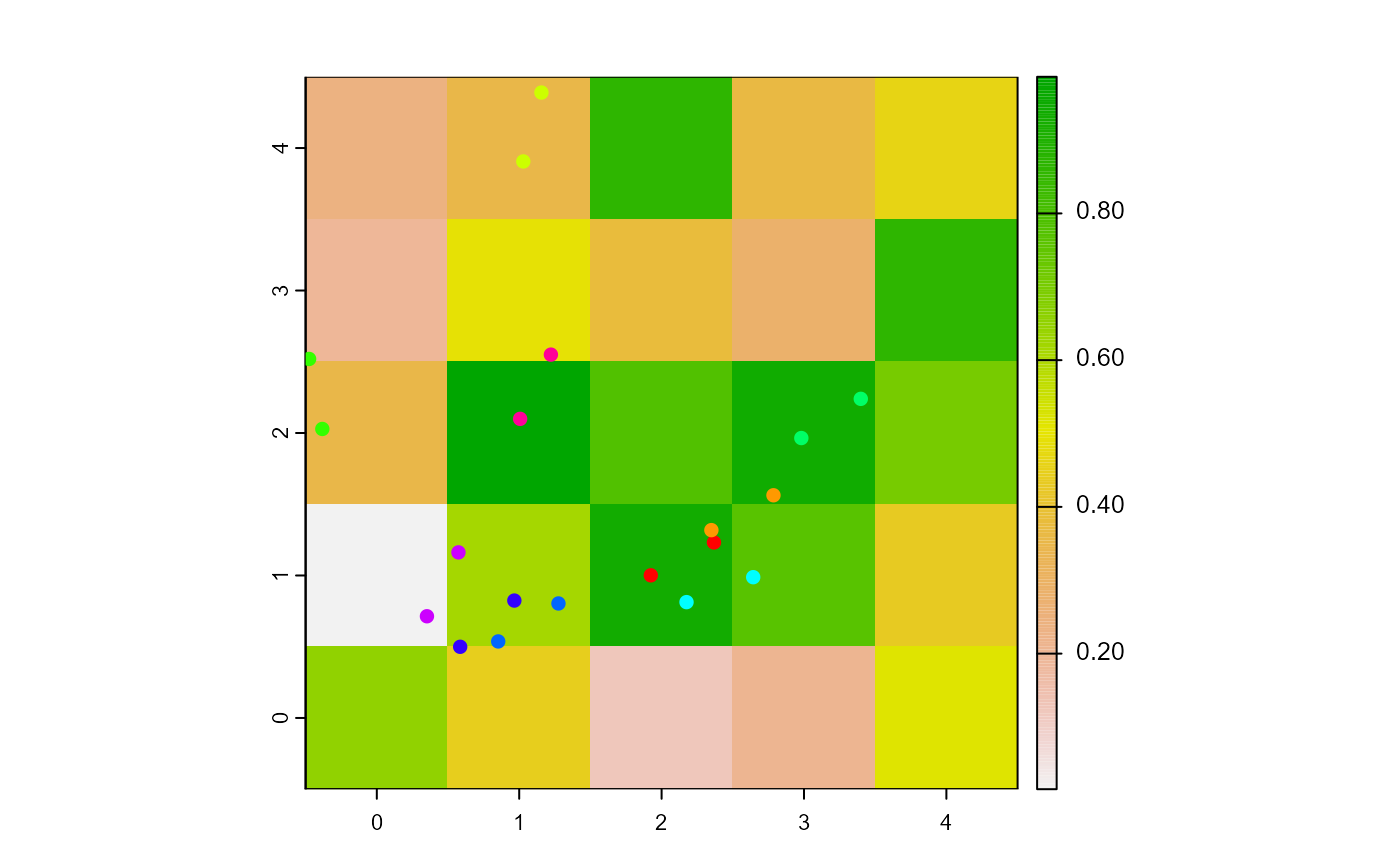Set the turtles' heading towards agents2.

face(turtles, agents2, world, torus = FALSE)

# S4 method for agentMatrix,matrix
face(turtles, agents2, world, torus = FALSE)

## Arguments

turtles

AgentMatrix object representing the moving agents.

agents2

Matrix (ncol = 2) with the first column pxcor and the second column pycor representing the patches coordinates, or

AgentMatrix object representing the moving agents, or

Matrix (ncol = 2) with the first column x and the second column y representing locations coordinates.

world

WorldMatrix or worldArray object.

torus

Logical to determine if the world is wrapped. Default is torus = FALSE.

## Value

AgentMatrix representing the turtles with updated headings.

## Details

The number of agents/locations in agents2 must be equal to 1 or to the length of turtles.

If torus = FALSE, world does not need to be provided.

If torus = TRUE and the distance from one turtles to its corresponding agent/location agents2 is smaller around the sides of the world than across it, then the direction to the agent/location agents2 going around the sides of the world is given to the turtle.

If a turtle is facing its own location, its heading does not change.

Sarah Bauduin

## Examples

w1 <- createWorld(minPxcor = 0, maxPxcor = 4, minPycor = 0, maxPycor = 4,
data = runif(25))
t1 <- createTurtles(n = 10, coords = randomXYcor(w1, n = 10))
plot(w1)
points(t1, col = of(agents = t1, var = "color"), pch = 16)

t1 <- face(turtles = t1, agents2 = cbind(x = 0, y = 0))
t1 <- fd(turtles = t1, dist = 0.5)
points(t1, col = of(agents = t1, var = "color"), pch = 16)